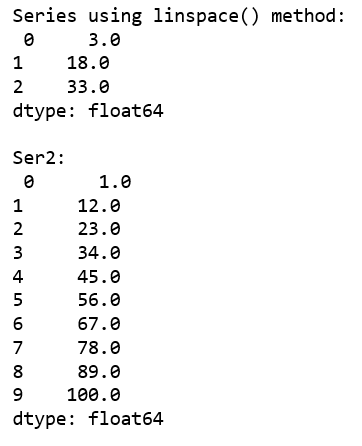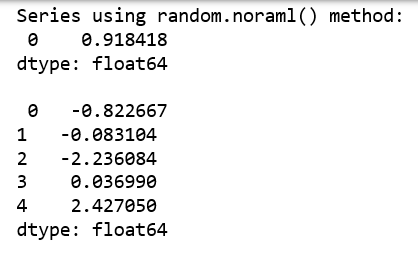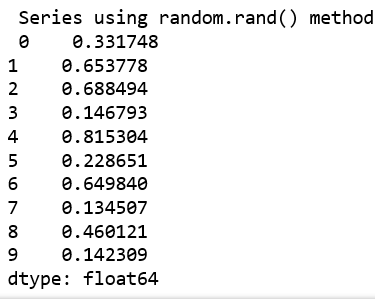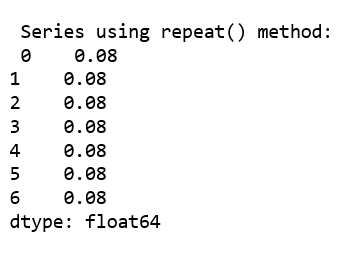# Create Pandas Series using NumPy functions

Pandas Series is a one-dimensional labeled array capable of holding data of any type (integer, string, float, python objects, etc.).

Let’s see how can we create a Pandas Series using different `numpy `functions.

Code #1: Using numpy.linspace()

 `# import pandas and numpy ` `import` `pandas as pd ` `import` `numpy as np ` ` `  `# series with numpy linspace()  ` `ser1 ``=` `pd.Series(np.linspace(``3``, ``33``, ``3``)) ` `print``(ser1) ` ` `  `# series with numpy linspace() ` `ser2 ``=` `pd.Series(np.linspace(``1``, ``100``, ``10``)) ` `print``(``"\n"``, ser2) ` ` `

Output:Code #2: Using np.random.normal() and random.rand() method.

 `# import pandas and numpy ` `import` `pandas as pd ` `import` `numpy as np ` ` `  `# series with numpy random.normal ` `ser3 ``=` `pd.Series(np.random.normal()) ` `print``(ser3) ` ` `  `# series with numpy random.normal ` `ser4 ``=` `pd.Series(np.random.normal(``0.0``, ``1.0``, ``5``)) ` `print``(``"\n"``, ser4) ` ` `  `# series with numpy random.rand ` `ser5 ``=` `pd.Series(np.random.rand(``10``)) ` `print``(``"\n"``, ser5) `

Output:Code #3: Using numpy.repeat()

 `# import pandas and numpy ` `import` `pandas as pd ` `import` `numpy as np ` ` `  ` `  `# series with numpy random.repeat ` `ser ``=` `pd.Series(np.repeat(``0.08``, ``7``)) ` `print``(``"\n"``, ser) `

Output:My Personal Notes arrow_drop_upCheck out this Author's contributed articles.

If you like GeeksforGeeks and would like to contribute, you can also write an article using contribute.geeksforgeeks.org or mail your article to contribute@geeksforgeeks.org. See your article appearing on the GeeksforGeeks main page and help other Geeks.

Please Improve this article if you find anything incorrect by clicking on the "Improve Article" button below.

Article Tags :

Be the First to upvote.

Please write to us at contribute@geeksforgeeks.org to report any issue with the above content.